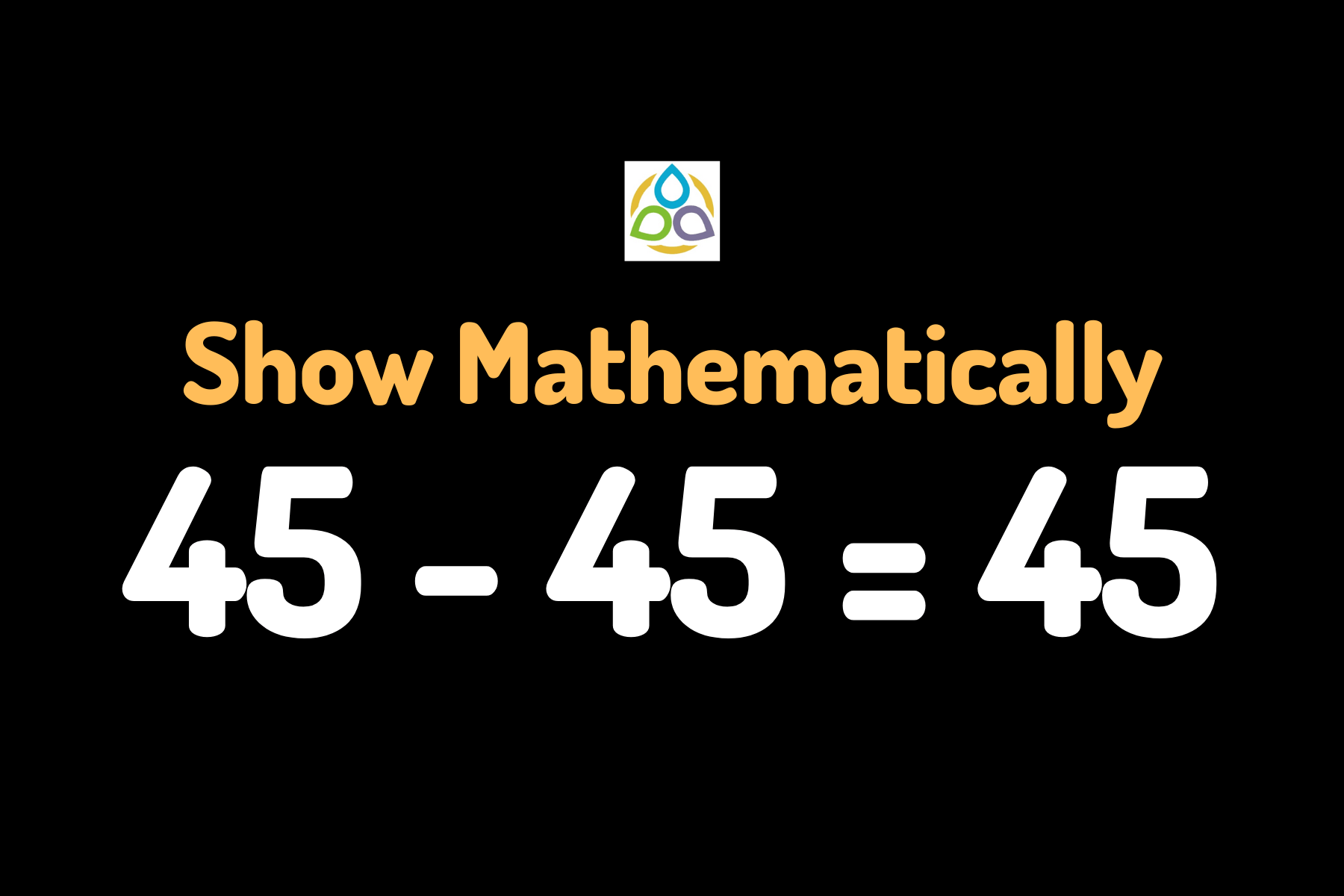# The Impossible Subtraction 45 – 45 = 45

For all their wealth of content, for all the sum of history and social institution invested in them, music, mathematics, and chess are resplendently useless (applied mathematics is a higher plumbing, a kind of music for the police band). They are metaphysically trivial, irresponsible. They refuse to relate outward, to take reality
for arbiter. This is the source of their witchery.

G. STEINER

Welcome to the blog Math1089 – Mathematics for All.

I’m glad you came by. I wanted to let you know I appreciate your spending time here on the blog very much. I do appreciate your taking time out of your busy schedule to check out Math1089!

Subtraction means taking away. Suppose you have 5 chocolates, and if 2 chocolates are taken away, then 3 chocolates will remain. Similarly, if 45 chocolates are taken away from a pack of 45 chocolates, then how come 45 chocolates will remain? As per the title of this blog post, we have 45 – 45 = 45! Hence the name Impossible Subtraction.

The present subtraction is not possible (in the known way); however, we have to make it possible. This means we have to think about it in a different way. What other way can we think of it? For example, we can think of two different numbers, 335 and 95. Why these two? Is there anything that is related to the present subtraction problem? Certainly! The digits of the numbers are 3, 3, 5 and 9, 5 respectively. Now, if we subtract the numbers, considering the product of the digits, we have

Clearly, our target is not being fulfilled. So, we have to still try it in a different way. Instead of a product, let us consider the sum of the digits. Hence, consider two numbers such that the sum of the digits of the numbers is 45 and the sum of the digits of the subtracted number is also 45. Thus the problem turns into a simple arrangement problem satisfying the given condition. Another difficult job!

For example, consider the numbers 333333333333333 and 555555555 having different numbers of digits. The sum of the digits of each number is 45, and the difference between the numbers is 333333333333333 – 555555555 = 333332777777778. But, the sum of the digits of this number = 3 + 3 + 3 + 3 + 3 + 2 + 7 + 7 + 7 + 7 + 7 + 7 + 7 + 7 + 8 = 81. Therefore, this is not acceptable.

Next, consider the numbers 555555555 and 99999 having different numbers of digits. The sum of the digits of each number is 45. Their difference is 555555555 – 99999 = 555455556 and the sum of the digits of this number = 5 + 5 + 5 + 4 + 5 + 5 + 5 + 5 + 6 = 45. Hence, this is a viable choice.

Finally, consider the numbers 987654321 and 123456789 having the same number of digits. Notice that the numbers are reverse of each other. The sum of the digits of each number is 45. Their difference is 987654321 – 123456789 = 864197532 and the sum of the digits of this number = 8 + 6 + 4 + 1 + 9 + 7 + 5 + 3 + 2 = 45. Hence, this is a viable choice.

Hence, the impossible subtraction turned into a possible subtraction!

Your suggestions are eagerly and respectfully welcome! See you soon with a new mathematics blog that you and I call Math1089 – Mathematics for All!“.